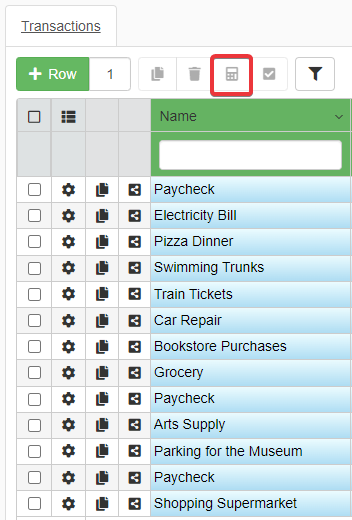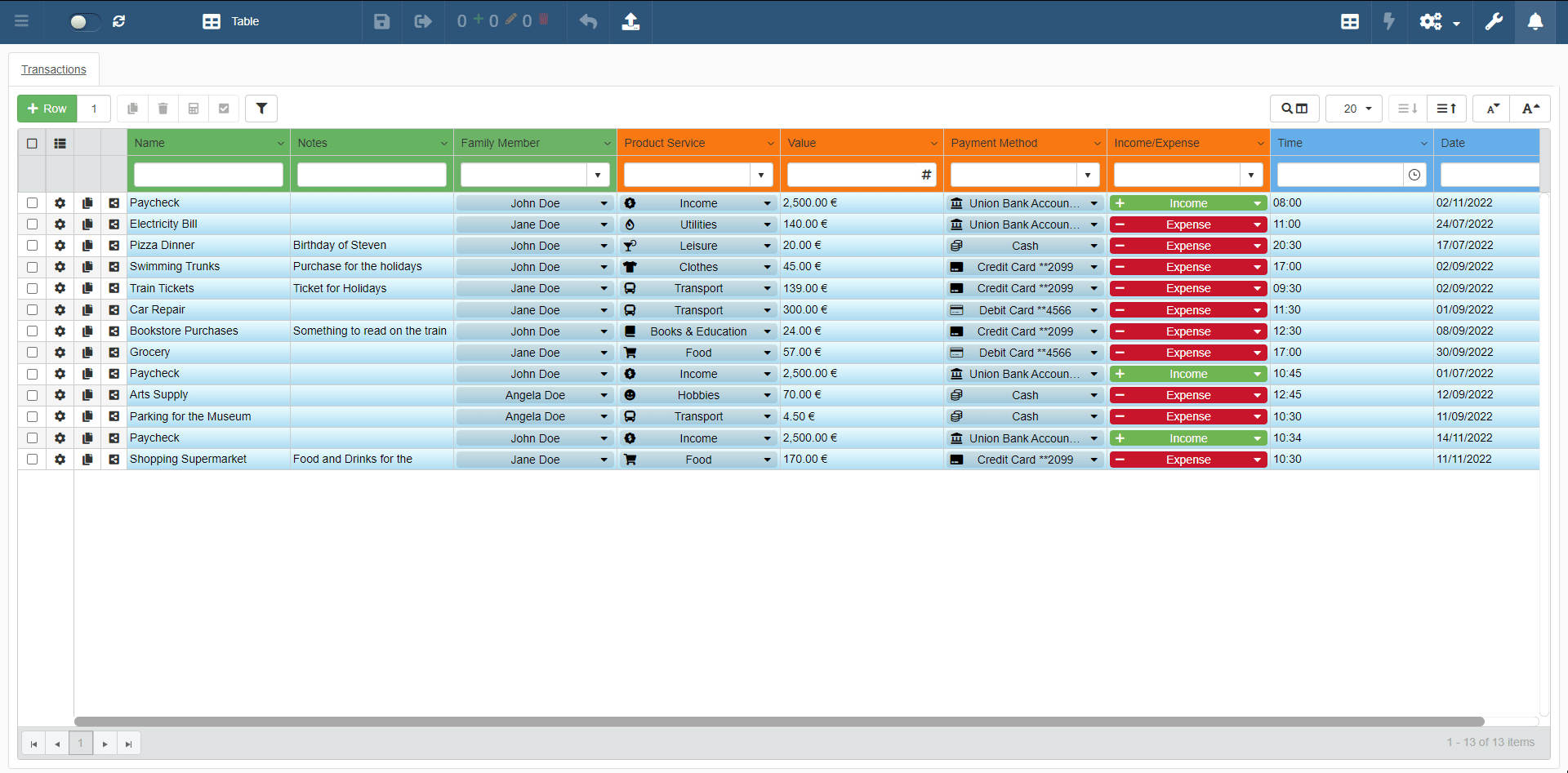# Recalculating Rows' Value

Learn how to recalculate one or more rows.

The Recalculate button () triggers the recalculation of calculated fields chosen by the Designer and relying on another application's information.

### Calculated Fields and Recalculation¶

Calculated Fields are column fields that obtain their values from a Formula. (See the Formulas section for more info.)

It is not necessary to understand how calculated fields work in detail. You must only know that the Designer can set up rules to calculate the value of one or more fields automatically.

Example

A calculated field calculating the total amount due including the VAT. The field updates automatically the value after a change. You don't need in this case the Recalculate.

In the example above, the application handles the recalculation. No action is required.

In other cases, a calculated field's value depends on values recorded in other applications.

Example

A calculated field calculating the total amount due including the VAT. The total amount depends on a list of services or products with a cost. The list of services and products is recorded through another application.

In the case above, the application doesn't update the field automatically since the values depends on an external source.

Use the Recalculate button () to recalculate the information relying on a different application according to the Designer's rules.

### How to Recalculate¶

You can recalculate a field by clicking on the Recalculate button ().

You can use the Recalculate button () to recalculate the calculated fields.1. Select the rows containing the cells you want to recalculate.
2. Click on the Recalculate button () in the upper-left corner of the table.The action recalculates the calculated values of the selected rows.

Note

The Designer configures what calculated cells you can recalculate within the application. So, the Recalculate button updates only certain values.

Note

The Designer decides to enable or disable the Recalculate action. The Designer might have either removed or limited the Recalculate action.